Graph IDE3D Data Graphics ► Point Map

A Point Map is a sequence of x-contiguous z-values arranged as a matrix that is mapped to a regular grid of cells. The z-value is mapped to the z-coordinate. The figures below show example point maps.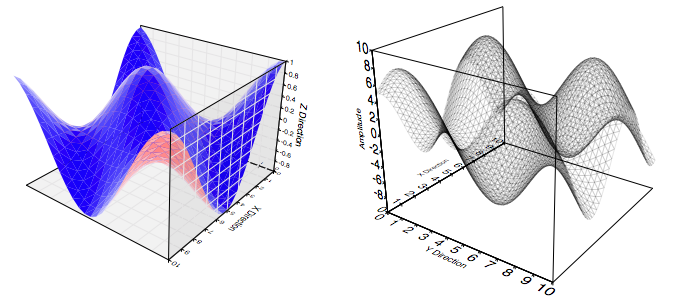Some standard operations are itemized below.

• To create a Point Map bring forward the Graphic Selector, click the Point Map factory cell and then mouse down on a Graphic View and drag the cursor to another point. The initial and final cursor locations define the boundary of a predefined Point Map graphic.

• You can also create 3D point maps from the standard Palettes menu item or drag them out from the 3D point map Factory Inspector.

• One thing you will probably want to do with a Point Map is modify its points. You can do that via the Data or Parser Inspector Editors.

• The Point Map conforms to the Color Map standards where the color map gradient is one of:

 Color Mapping Type No Color Map The color map is off. This makes most sense while just drawing the stroke so that only the cell boundaries appear. False Color The cell color is mapped from arbitrary independent color values inserted into the color table. Notice that a cell is a triangular region that is interpolated from the nearest z-values in the data sequence. Radiance The color is mapped based on the angle between the surface normal and the viewing angle. Note that the colors start at negative curl and proceed to positive curl, i.e.: The underside of a surface is the start of the color map and the overside is the end. Z-Value The color is mapped from the z-value of the surface. This mapping is very common, albeit somewhat redundant because the surface z-values are already represented by 3d graph although a color mapping of this type is more quantitative because the projection mapping is not present in the color definition. Distance The color is mapped from a value related by the distance of the cell to the viewer. The distance is normalized so that the nearest and farthest points of surface define the distance extremum.

• To program a 3D point map see the Programming section.

Data Editor

The Data Editor for the Point Map is shown below.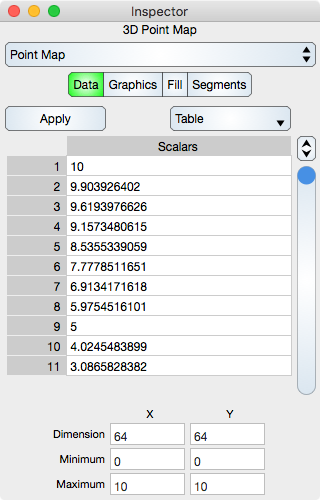Table

Apply : Once the grid values have been entered then click the Apply button to redefine the grid.

Table controls are described in the Tables section.

The rows represent the z-value of the regular grid.

Grid Values

The data table does not specify grid parameters, it only specifies amplitude values. That is because a point fill map is on a regular grid. A regular grid is defined by the six values described below.

Dimension : The number of cells in the x and y dimension, aka: The dimensions of the matrix of values. The product of x and y dimensions should equal the number of rows in the table.

Minimum : The x and y minimum of the grid.

Maximum : The x and y maximum of the grid.

Notice that cell center points, which is also considered where the data is on the grid, are coincidental with the grid minimum and maximum. As a result, the cells along the boundary are actually half cells and at the corners quarter cells. This is a very slight but important distinction.

Graphics Editor

The Graphics Editor for the Point Map is shown below.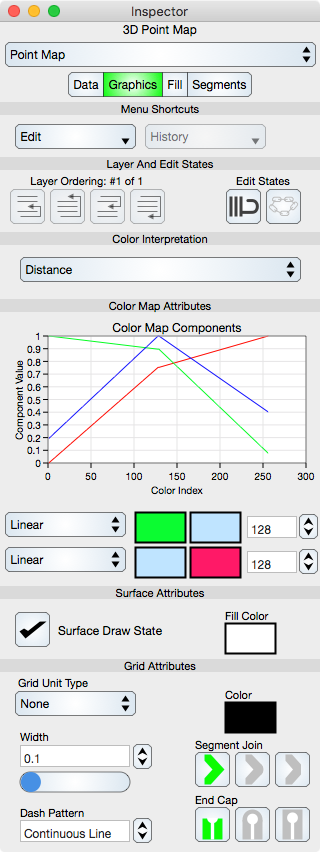Common Controls

Controls common to all graphics are described in the Graphics section.

Color Map Attributes

The color map is further described in the Color Map section.

Graph : Shows a graph of the color map.

Function : Select this to set the color map function. Typically it is set to linear.

Start Color : Defines the start Color of the color map.

End Color : Defines the end Color of the color map.

Number : Defines the number of samples in the color map.

Surface Attributes

Surface Draw State : If checked then the surface is drawn.

Fill Color : Defines the fill Color for the surface. This is normally overridden by the Color Map.

Grid Attributes

Grid attributes are controlled by the normal stroke controls defined in the Graphics section.

Fill Editor

The Fill Editor for the 3D Point Fill graphic is shown below.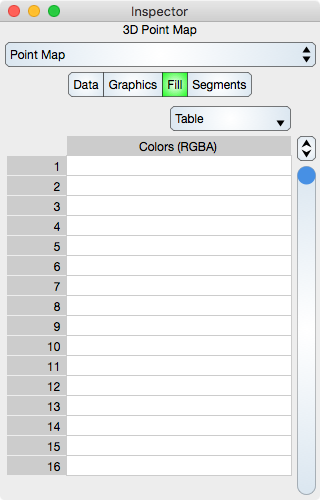Fill attributes is a false color table of RGBA components, one for each cell. These can be overridden by the Color Interpretation setting and that setting must be False Color Map in order for the elements of this table to take effect.

Table

Table controls are described in the Tables section.

The rows represent a segment color values. While in Atomic mode, the cell represents a rgba value and while in component mode the cell represents either single r, g, b and a. Hence, in atomic mode there is one column while in component mode there are four columns.

Note that if the Data sequence is changed then the Fill sequence will not change.

Segments Editor

The Segments Editor for the 3D Point Fill graphic is shown below.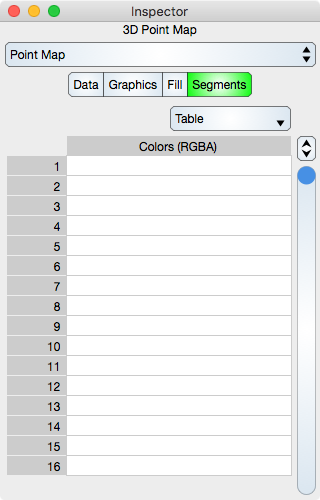Segments are the line segments of the grid. See Sequence Colors for additional information. If the diagonal grid line segments are given a transparent color then the grid tessellation can appear to show x or y curves along the surface and those curves can have a defined color mapping and thus show another dimension of the data.

Table

Table controls are described in the Tables section.

The rows represent a segment color values. While in Atomic mode, the cell represents a rgba value and while in component mode the cell represents either single r, g, b and a. Hence, in atomic mode there is one column while in component mode there are four columns.

Note that if the Data sequence is changed then the Segments sequence will not be change.